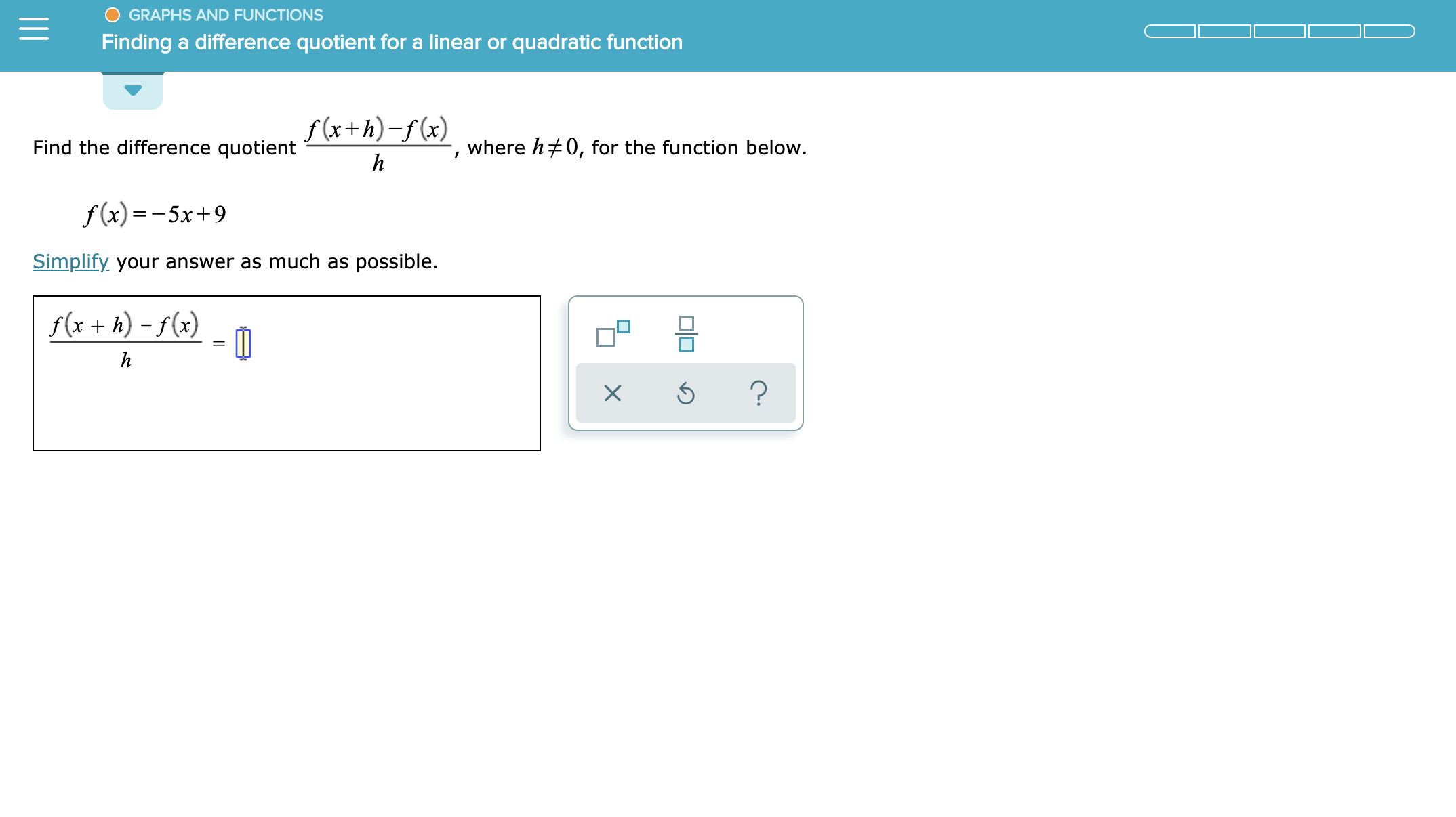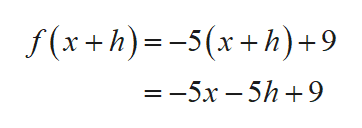GRAPHS AND FUNCTIONSFinding a difference quotient for a linear or quadratic functionf (x+h)-f(x)where h0, for the function below.Find the difference quotienthf(x)-5x 9Simplify your answer as much as possible.f(x +h)-f(x)h?X

Question

see attachmenthelp_outlineImage TranscriptioncloseGRAPHS AND FUNCTIONS Finding a difference quotient for a linear or quadratic function f (x+h)-f(x) where h0, for the function below. Find the difference quotient h f(x)-5x 9 Simplify your answer as much as possible. f(x +h)-f(x) h ? X fullscreen
Step 1

Consider the given function:

Step 2

Write the formula for difference quotient:

Step 3help_outlineImage Transcriptionclosef (x+h)-5x+h)+9 — — 5х —5h +9 fullscreen

Want to see the full answer?

See Solution

Want to see this answer and more?

Our solutions are written by experts, many with advanced degrees, and available 24/7

See Solution
Tagged in

Calculus